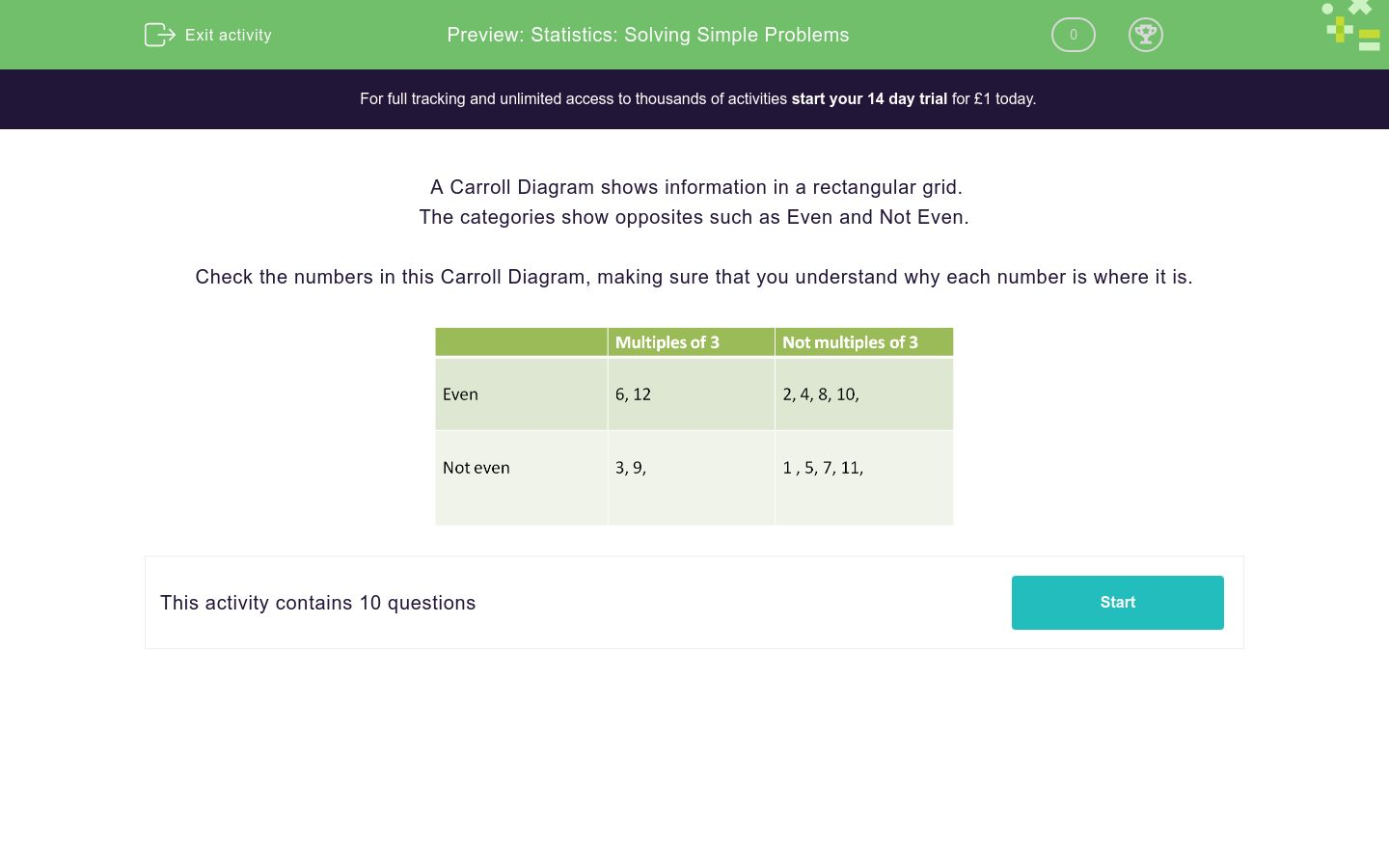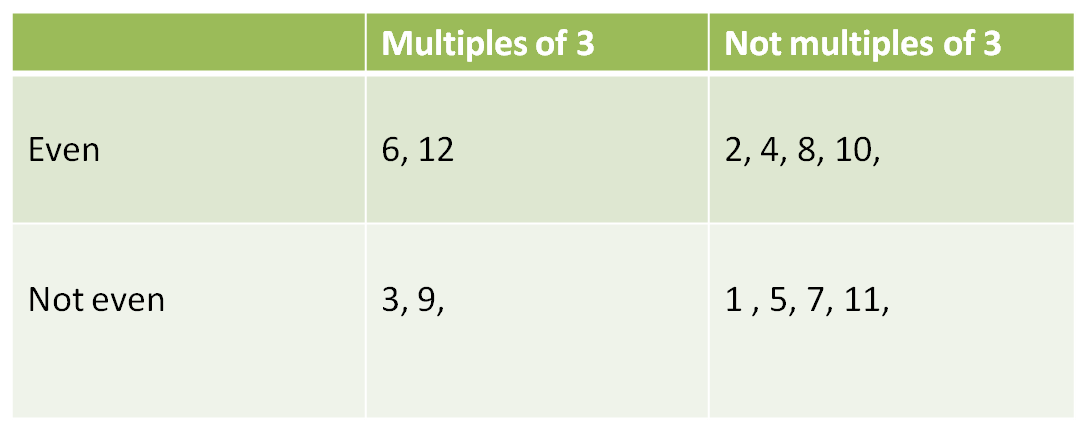# Statistics: Solving Simple Problems

In this worksheet, students answer questions based on a diagram.Key stage:  KS 2

Curriculum topic:   Statistics

Curriculum subtopic:   Solve Statistic Problems

Difficulty level:### QUESTION 1 of 10

A Carroll Diagram shows information in a rectangular grid.

The categories show opposites such as Even and Not Even.

Check the numbers in this Carroll Diagram, making sure that you understand why each number is where it is.Use this Carroll Diagram to select all the even multiples of 3 between 1 and 12.1

2

3

4

5

6

7

8

9

10

11

12

Use this Carroll Diagram to select all the odd multiples of 3 between 1 and 12.1

2

3

4

5

6

7

8

9

10

11

12

Use this Carroll Diagram to select all the odd numbers which are not multiples of 3 between 1 and 12.1

2

3

4

5

6

7

8

9

10

11

12

Use this Carroll Diagram to select all the even numbers which are not multiples of 3 between 1 and 12.1

2

3

4

5

6

7

8

9

10

11

12

In which section does the number 5 go in this Carroll Diagram?A

B

C

D

In which section does the number 12 go in this Carroll Diagram?A

B

C

D

In which section does the number 15 go in this Carroll Diagram?A

B

C

D

In which section does the number 30 go in this Carroll Diagram?A

B

C

D

In which section does the number 33 go in this Carroll Diagram?A

B

C

D

In which section does the number 1 go in this Carroll Diagram?A

B

C

D

• Question 1

Use this Carroll Diagram to select all the even multiples of 3 between 1 and 12.6
12
EDDIE SAYS
The first box in the Carroll Diagram shows that the even multiples of 3 are 6 and 12.
• Question 2

Use this Carroll Diagram to select all the odd multiples of 3 between 1 and 12.3
9
EDDIE SAYS
In the Carroll Diagram it shows the odd (not even) multiples of 3 are 3 and 9.
• Question 3

Use this Carroll Diagram to select all the odd numbers which are not multiples of 3 between 1 and 12.1
5
7
11
EDDIE SAYS
In the Carroll Diagram it shows which odd (not even) numbers are not multiples of 3: 1, 5, 7, 11.
• Question 4

Use this Carroll Diagram to select all the even numbers which are not multiples of 3 between 1 and 12.2
4
8
10
EDDIE SAYS
In the Carroll Diagram it shows the even numbers that aren't multiples of 3: 2, 4, 8, 10.
• Question 5

In which section does the number 5 go in this Carroll Diagram?C
EDDIE SAYS
The number 5 is an odd multiple of 5, so it will go in section C.
• Question 6

In which section does the number 12 go in this Carroll Diagram?B
EDDIE SAYS
12 goes in section B because it's even and not a multiple of 5.
• Question 7

In which section does the number 15 go in this Carroll Diagram?C
EDDIE SAYS
15 goes in section C because it's an odd multiple of 5.
• Question 8

In which section does the number 30 go in this Carroll Diagram?A
EDDIE SAYS
30 goes in section A because it's an even multiple of 5.
• Question 9

In which section does the number 33 go in this Carroll Diagram?D
EDDIE SAYS
33 goes in section D because it's odd and not a multiple of 5.
• Question 10

In which section does the number 1 go in this Carroll Diagram?D
EDDIE SAYS
1 goes in section D because it's odd and not a multiple of 5. Well done!
---- OR ----

Sign up for a £1 trial so you can track and measure your child's progress on this activity.

### What is EdPlace?

We're your National Curriculum aligned online education content provider helping each child succeed in English, maths and science from year 1 to GCSE. With an EdPlace account you’ll be able to track and measure progress, helping each child achieve their best. We build confidence and attainment by personalising each child’s learning at a level that suits them.

Get started